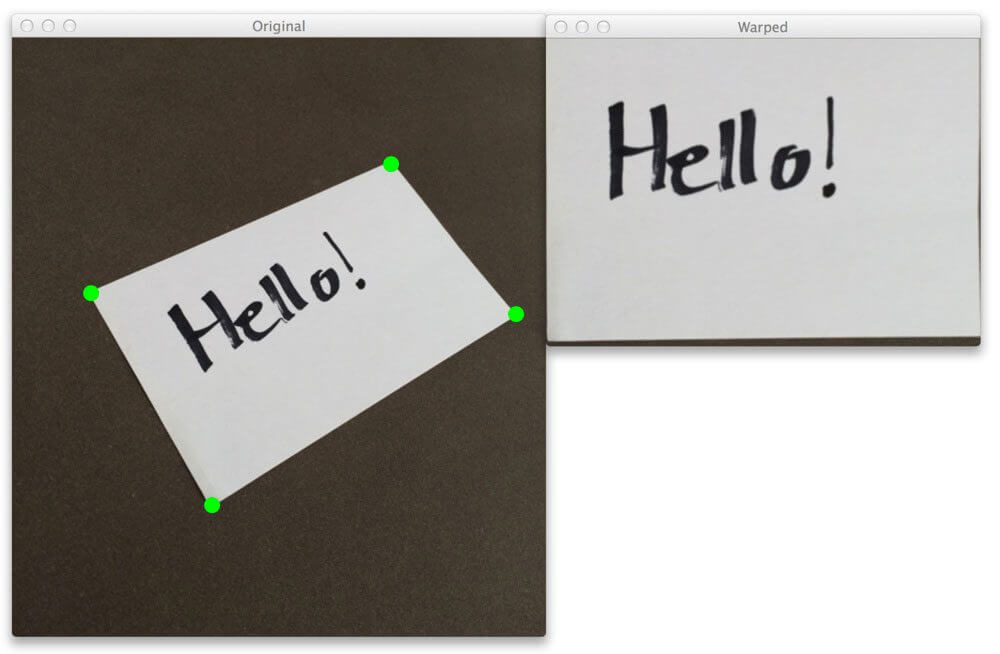Notebook

# Digital Image Processing

In :
% run load_lib_ch5.py


## Chapter 5: Geometric Transformations

Geometric transformations are image operations that modify the positions of the pixels in an image but keep the pixel values unchanged. In this chapter only linear operations will be discussed. These operations include rotation, scaling and translation, they can be understood as a mapping of a 2D-plane in 3D-space to another 2D-plane.### Camera Projection

#### Extrinsic Projection

Imagine a camera pointing at an object. The camera projects the points of the object onto it's own camera coordinates. For example, if the camera was put upside down, the object relative to the ground would not change, but the object that the camera sees does. This can be shown with the following transformation matrix where $R$ is a 3D rotation and $t$ is a 3D displacement. It deals with the camera's extrinsics.

$\begin{bmatrix} & & & t_x\\ & R_{3x3} & & t_y\\ & & & t_z \end{bmatrix} = \begin{bmatrix} R_{3x3} & \vec{t} \end{bmatrix}=M_{affine}$

This transformation matrix has 6 degrees of freedom (DoF) - it can manipulate displacement in x,y and z directions and also rotation relative to x,y and z axes.

$\begin{bmatrix} x' \\ y' \\ z' \\ 1 \end{bmatrix} = M_{affine} \begin{bmatrix} x \\ y \\ z \\ 1 \end{bmatrix}$

#### Intrinsic Projection

After that the 3D objects that the camera sees need to be projected onto a 2D plane. In order to do this we need to get rid of the z-coordinate. This is done with the following normalized z mapping where $f$ is the focal length or scale or simply, how close the camea plane is to the camera pinhole sensors. It deals with the camera's intrinsics.

$x'= f\cfrac{x}{z}$,

$y' = f\cfrac{y}{z}$

Also shown as:

$\begin{bmatrix} x' \\ y' \\ 1 \\ 1 \end{bmatrix} = \begin{bmatrix} f & 0 & 0\\ 0 & f & 0\\ 0 & 0 & 1\\ \end{bmatrix} \begin{bmatrix} x \\ y \\ z \\ 1 \end{bmatrix} = M_{projective} \begin{bmatrix} x \\ y \\ z \\ 1 \end{bmatrix}$

### Homography

The affine and projective matrices are combined together to form a homography matrix that contains all the parameters. World coordinate z is assumed as 0.

$M_{projective}.M_{affine} \begin{bmatrix} x \\ y \\ 0 \\ 1 \end{bmatrix} = \begin{bmatrix} f & 0 & 0 \\ 0 & f & 0 \\ 0 & 0 & 1 \\ \end{bmatrix} \begin{bmatrix} & & t_x\\ & R_{3x2} & t_y\\ & & t_z \end{bmatrix} \begin{bmatrix} x \\ y \\ 1 \end{bmatrix}=$

$= \begin{bmatrix} f.r_{11} & f.r_{12} & f.t_x\\ f.r_{21} & f.r_{22} & f.t_y\\ r_{31} & r_{32}& t_z \end{bmatrix} \begin{bmatrix} x \\ y \\ 1 \end{bmatrix} = H \begin{bmatrix} x \\ y \\ 1 \end{bmatrix}$

A homography is a matrix that has 9 parameters. For a projected point $p$ as $H.p=p'$, it is also true that $c.H.p = c.p'$. Therefore, the scaling $c$ does not affect the final result for $p'$. Therefore a homography has 8 degrees of freedom instead of 9.

### Euclidian Transform

Euclidian transformations are ones that preserve the parallel lines and theyr lengths. They have 3 degrees of freedom - rotation along z-axis and translation in x and y directions. The formula is generalized for $f=1$, $t_z=1$, $r_{31}$ and $r_{32}=0$.

$s.\begin{bmatrix} u \\ v \\ 1 \end{bmatrix}$ = $\begin{bmatrix} x \\ y \\ 1 \end{bmatrix} \begin{bmatrix} R_{2x2} & & t_x\\ & & t_y\\ 0 & 0 & 1 \end{bmatrix}$

#### Translation

When there is no rotation, the rotation matrix $R_{2x2}$ is equal to the identity matrix $I_{2x2}$. $tx$ and $ty$ allow for a translation in x- and y-directions.

$H= \begin{bmatrix} 1 & 0 & t_x\\ 0 & 1 & t_y\\ 0 & 0 & 1 \end{bmatrix}$

In :
tx = 50
ty = 60
M = np.float32([
[1,0,tx],
[0,1,ty],
[0,0, 1]])

In :
apply_geometric_trans(img, M)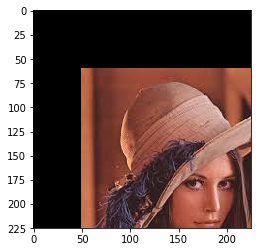#### Rotation

When there is no translation, the translation matrix is equal to 0. $R$ allows for a rotation with angle $\theta$ with respect to (x,y)=(0,0).

$H= \begin{bmatrix} R_{2x2} & & 0\\ & & 0\\ 0 & 0 & 1 \end{bmatrix} = \begin{bmatrix} \cos{\theta} & -\sin{\theta} & 0\\ \sin{\theta} & \cos{\theta} & 0\\ 0 & 0 & 1 \end{bmatrix}$

In :
theta_deg = 25

M = np.float32([
[c,-s,0],
[s, c,0],
[0, 0,1]])

In :
apply_geometric_trans(img, M)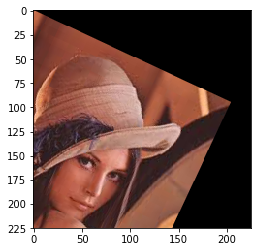The translation and rotation can be combined to change the axis of rotation to a different (x,y) coordinate.

In :
theta_deg = 25

M = np.float32([
[c,-s,50],
[s, c,-40],
[0, 0,1]])

In :
apply_geometric_trans(img, M)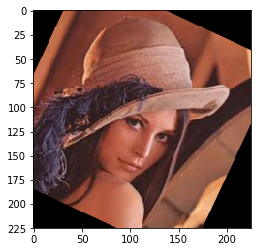### Similarity Transform

Similarity transformations have 4 DoF - on top of Euclidian transformations they also use the scale parameter $s$. They take the form $\begin{bmatrix} s.r_{11} & r_{12} & t_x\\ r_{21} & s.r_{22} & t_y\\ 0 & 0 & 1 \end{bmatrix}$

In :
M = np.float32([
[1.3,  0,0],
[  0,1.3,0],
[  0,  0, 1]])

In :
apply_geometric_trans(img, M)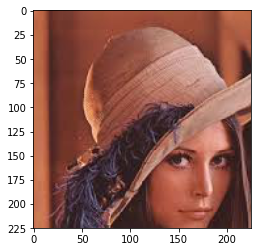### Affine Transform

Affine transforms have 6 DoF. Unlike the similarity or euclidian transforms, in affine transforms the parameters $r_{n}$ are independent of eachother. It has 6 parameters that control scale spect ratio, orientation, displacement (x/y) and shear (x/y). The final matrix takes the form $\begin{bmatrix} a_{11} & a_{12} & t_x\\ a_{21} & a_{22} & t_y\\ 0 & 0 & 1 \end{bmatrix}$. Affine transfomrations preserve the parallel lines but not their length.

In :
M = np.float32([
[0.8, -0.1,20],
[0, 0.8,20],
[0, 0,1]])

In :
apply_geometric_trans(img, M)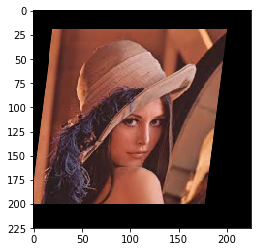### Pespective Transform

The final transfomration is the perspective transformation which preserves lines but not their parallelity or length. To achieve that a homography matrix is used. It has 8 DoF $H = \begin{bmatrix} h_{11} & h_{12} & h_{13}\\ h_{21} & h_{22} & h_{23}\\ h_{31} & h_{32} & h_{33} \end{bmatrix}$. The perspective transform can be computed by solving the desired equation. Check out more about homography estimation here. More about perspective transformation will be shown in the image stitching chapter.